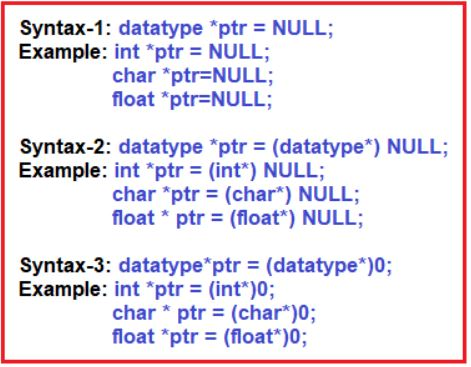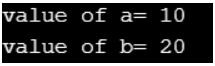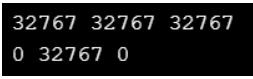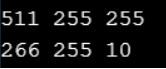# Null Pointer in C

## Null Pointer in C Language with Examples

In this article, I am going to discuss Null Pointer in C Language with Examples. Please read our previous articles, where we discussed Pointer to Constant in C Language with Examples.

##### What is a Null Pointer?

A Null Pointer in C Programming Language is a pointer that does not point to any memory location i.e. it does not hold the address of any variables. It only stores the base address of the segment. That means the null pointer in C stores the Null value while the void is the type of the pointer. A null pointer is a special reserved value that is defined in a stddef header file. Here, Null means that the pointer is referring to the 0th memory location.

If we do not have any address which is to be assigned to the pointer, then it is known as a null pointer. When a NULL value is assigned to the pointer, then it is considered a Null pointer. So, A null pointer is a pointer that points to nothing. Some uses of the null pointer are as follows:

• Used to initialize a pointer variable when that pointer variable isn’t assigned any valid memory address yet.
• Used to pass a null pointer to a function argument when we don’t want to pass any valid memory address.
• Used to check for a null pointer before accessing any pointer variable. So that, we can perform error handling in pointer-related code e.g. dereference pointer variable only if it’s not NULL.
##### Null Pointer in C Language:

The pointer variable which is initialized with the null value is called the Null Pointer. Null Pointer doesn’t point to any memory location until we are not assigning the address. The size of the Null pointer also is 2 bytes according to DOS Compiler.The null pointer can be used as an error code of a function. Null can be used as a constant integral value.

Example: int x=NULL; //int x=0;

By using NULL, we can check the status of a resource, i.e. it is busy with any process or free to utilize.

##### Program to Understand Null Pointer in C Language:
```#include<stdio.h>
void main ()
{
int a,b;
int *ptr = (int *) 0;
if (ptr == 0)
{
ptr = &a;
a = 100;
}
if (ptr == (int *) 0)
{
ptr = &b;
b = 200;
}
printf ("value of *ptr: %d ", *ptr);
}
```

Output: value of *ptr: 100

##### What will be the output in the below program?
```#include<stdio.h>
int main ()
{
int a, b;
int *ptr = (int *) 0; //int *ptr = NULL;
if (ptr == (int *) 0)
{
ptr = &a;
a = 10;
printf ("value of a= %d", *ptr);
ptr = (int *) 0;
}
if (ptr == NULL)
{
ptr = &b;
b = 20;
printf ("\nvalue of b= %d", *ptr);
}
return 0;
}
```
###### Output:##### What will be the output from the below program?
```#include<stdio.h>
int main ()
{
int a, b;
int *ptr = (int *) NULL;
ptr = &a;
a = 32767;
b = *ptr;
printf ("\n %d %d %d", a, b, *ptr);
*ptr = 0;
printf ("\n %d %d %d", a, b, *ptr);
return 0;
}
```
###### Output:##### What will be the output from the below program?
```#include<stdio.h>
void main ()
{
int a, b;
unsigned char *ptr = (unsigned char *) 0;
ptr = &a;
a = 511;
b = *ptr;
printf ("%d %d %d \n", a, b, *ptr);
*ptr = 10;
printf ("%d %d %d", a, b, *ptr);
}
```
###### Output:Note: On integer variable when we are applying an unsigned character pointer then it can access and manipulate 1byte data only because indirection operator behavior is datatype dependent.

#### Null Pointer use Cases in C Language:

##### When we do not assign any memory address to the pointer variable.

In the below example, we declare the pointer variable *ptr, but it does not contain the address of any variable. The dereferencing of the uninitialized pointer variable will show the compile-time error as it does not point to any variable. The following C program shows some unpredictable results and causes the program to crash. Therefore, we can say that keeping an uninitialized pointer in a program can cause the program to be crashed.

```#include <stdio.h>
int main()
{
int *ptr;
printf ("Address: %d", ptr); // printing the value of ptr.
printf ("Value: %d", *ptr);	// dereferencing the illegal pointer
return 0;
}```
###### How to avoid the above problem?

In C Programming Language, we can avoid the above problem by using a Null pointer. A null pointer is a pointer pointing to the 0th memory location, which is a reserved memory and cannot be dereferenced. In the below example, we create a pointer *ptr and assign a NULL value to the pointer, which means that it does not point to any variable. After creating a pointer variable, we add the condition in which we check whether the value of a pointer is null or not.

```#include <stdio.h>
int main()
{
int *ptr = NULL;
if (ptr != NULL)
{
printf ("value of ptr is : %d", *ptr);
}
else
{
printf ("Invalid pointer");
}
return 0;
}```
##### When we use the malloc() function?

In the below example, we use the built-in malloc() function to allocate the memory. If the malloc() function is unable to allocate the memory, then it returns a NULL pointer. Therefore, it is necessary to add the condition which will check whether the value of a pointer is null or not, if the value of a pointer is not null means that the memory is allocated.

```#include <stdio.h>
int main()
{
int *ptr;
ptr = (int *) malloc (5 * sizeof (int));
if (ptr == NULL)
{
printf ("Memory is not allocated");
}
else
{
printf ("Memory is allocated");
}
return 0;
}```

Note: It is always a good programming practice to assign a Null value to the pointer when we do not know the exact address of memory.

##### Applications of Null Pointer

Following are the applications of a Null pointer:

• It is used to initialize the pointer variable when the pointer does not point to a valid memory address.
• It is used to perform error handling with pointers before dereferencing the pointers.
• It is passed as a function argument and to return from a function when we do not want to pass the actual memory address.